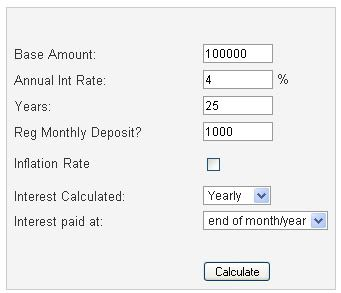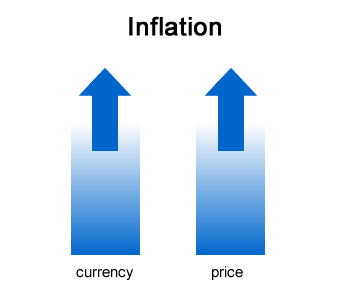# Nominal vs Real Interest Rates

A nominal rate of return is also called gross rate of return. The real rate of return is simply the gross return less inflation. Do you think is it better to use real returns or nominal returns for your future projections?Due to the inflation changes the money value, so the investment value of RM100,000 today is totally different it was 20 years ago.

When I plan for my retirement savings, I always use real rates of return with an inflation rate of x%. The reason is to make the numbers more understandable. Let’s take an example:

Michael and Jackson are 30 years old and want to retire in next 25 years. Let say they own RM100,000 in investments and are able to put away RM1000 per month. If we assume a nominal rate of return of 8% and an inflation rate of 4%, how much they will have at retirement age?

# How to Calculate Future Value of Money Using Inflation Rates?

I believe that most of the people worry about the inflation. Inflation not only can increase the cost of goods but also able to drag down your living standard. In the other words, it causes our money less value and decreases our purchasing power. You can buy less thing with RM5 today compare to ten years ago. We can have two plates of mee with RM5 ten years ago but today we can buy less than two plates. So, this is inflation! As a financial planner, they should know how to calculate the future possible inflation rates and advise investments that able to beat inflation.As an individual, it’s better to know how to calculate your inflation adjusted expense in the future. If your current expense is RM1,000 per month, in the future, let say after 20 years, an expected inflation rate of 5% or 7%, then how can you calculate the amount required to bear for each month expenses at that time?

Here is my simple & effective calculation method that you can use to calculate the total amount you required each month after 20 years and expected inflation rate is 7%.

The formula is = Current Amount x (1+inflation%) ^ Number of Years

So, let say you have a current monthly expense RM1,000 and after 20 years, it would be: